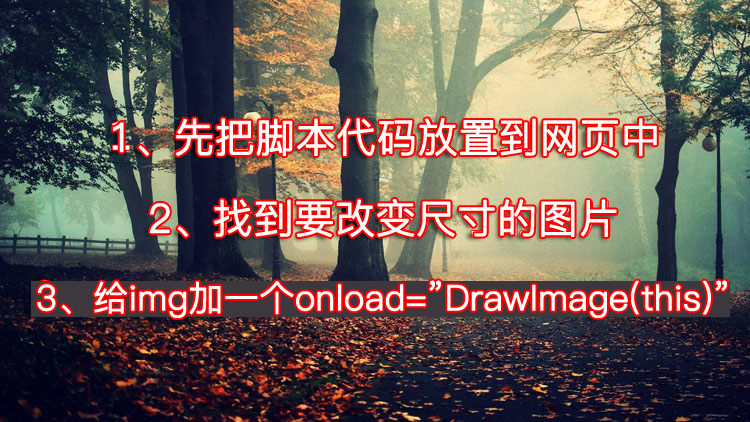<script language="JavaScript" type="text/JavaScript">
function DrawImage(ImgD){
var image=new Image();
var iwidth = 380; //当宽度大于380px时等比例缩小
var iheight = 500; //定义允许图片高度，当高度大于500px时等比例缩小
image.src=ImgD.src;
if(image.width>0 && image.height>0){
flag=true;
if(image.width/image.height>= iwidth/iheight){
if(image.width>iwidth){
ImgD.width=iwidth;
ImgD.height=(image.height*iwidth)/image.width;
}else{
ImgD.width=image.width;
ImgD.height=image.height;
}
ImgD.alt=image.width+"×"+image.height;
}
else{
if(image.height>iheight){
ImgD.height=iheight;
ImgD.width=(image.width*iheight)/image.height;
}else{
ImgD.width=image.width;
ImgD.height=image.height;
}
ImgD.alt=image.width+"×"+image.height;
}
}
}</script>